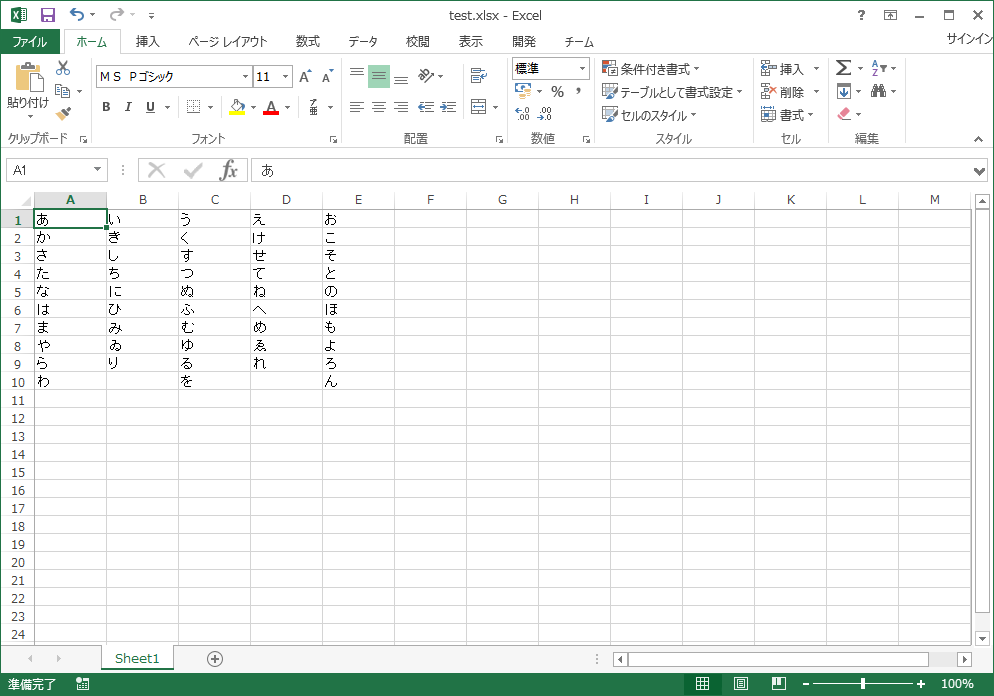# Introduction

This time is Apache POI. I used it to import the data group created by Excel into Java processing.

## What is Apache POI

Official name Apache POI Java library that can read and write Microsoft Office format files such as Word and Excel

• See Wiki https://ja.wikipedia.org/wiki/Apache_POI

poi-4.1.1.jar poi-ooxml-4.1.1.jar poi-ooxml-schemas-4.1.1.jar commons-collections4-4.4.jar commons-compress-1.19.jar xmlbeans-3.1.0.jar

I made a process to import the first sheet of the target Excel file, column A, into Java.

#### `SamplePOI.java`

``````
public class SamplePOI {

/*
*This process
*/
public static void main(String[] args) {
//Enter the full path of the Excel file you want to import here
String ExcelPath = "";

//Objects for Excel
Workbook wb;
Sheet sh;
Row row;
Cell cell;

//List to hold the acquired data
List<String> columnA_List = new ArrayList<>();

try (InputStream is = new FileInputStream(ExcelPath)) {

//Import the target Excel file into Java
wb = WorkbookFactory.create(is);
//Specify the first sheet of the target file
sh = wb.getSheetAt(0);
//Get the maximum row in the sheet
int rowMaxA = sh.getLastRowNum();

//Turn the loop for the maximum row and get the cell of column A as a String type
for (int i = 0; i <= rowMaxA; i++) {
row = sh.getRow(i);
cell = row.getCell(0);
String cellValue = getCellStringValue(cell);
}

//Output to console
System.out.print("[");
for (String outStr : columnA_List) {
System.out.print(outStr);
}
System.out.print("]");

} catch (Exception e) {
e.printStackTrace();
}
}

/*
*Determines the state of the cell and returns it as a String type.
*/
private static String getCellStringValue(Cell cell) {
String retStr;
CellType cellType = cell.getCellType();
switch (cellType) {
case STRING:
retStr = cell.getStringCellValue();
break;
case NUMERIC:
retStr = String.valueOf(cell.getNumericCellValue());
break;
case BOOLEAN:
retStr = String.valueOf(cell.getBooleanCellValue());
break;
case FORMULA:
retStr = String.valueOf(cell.getCellFormula());
break;
case ERROR:
retStr = String.valueOf(cell.getErrorCellValue());
break;
default:
retStr = "";
break;
}
return retStr;
}
}
``````

## Try to run

When I load the Excel file containing the following hiragana and execute it, "Akasatana Hamayarawa" is output.#### `samplePOI.result`

``````
[a Ka sa ta na ha ma ya ra wa]
``````

# Summary

I only did simple processing, but I felt that it was the same as VBA. It was a little difficult to understand that the cell type had to be taken into consideration when acquiring the contents of the cell.

When I try to insert a function in Excel and import it, it seems that the function itself is acquired as a value instead of the calculation result? So if you want the result, it seems a little difficult.